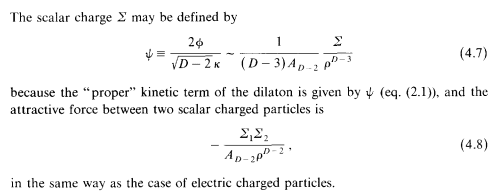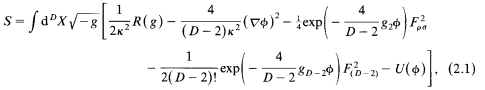# Question about dilaton monopole interaction derivation

• A
• user1139

#### user1139

TL;DR Summary
I am trying to understand how one derives the dilaton monopole interaction. In "Black holes and membranes in higher-dimensional theories with dilaton fields", Gibbons and Maeda mentioned that one could obtain the dilaton monopole interaction as such:where the action is given byHowever, I do not understand their reasoning for introducing ##\Psi## to define ##\Sigma## in order to derive Eq. (4.8). Could someone explain it?

If you look at the coefficient of (∇φ)2 in their equation 2.1, you'll see it's minus the square of the field redefinition factor. So they must be aiming for a dilaton kinetic term with a coefficient of 1.

If you look at the coefficient of (∇φ)2 in their equation 2.1, you'll see it's minus the square of the field redefinition factor. So they must be aiming for a dilaton kinetic term with a coefficient of 1.
Still, how do they get ##\Sigma## from ##\Psi##? Did they just consider the asymptotic behaviour of ##\Psi## and define ##\Sigma## as such?

4.7, 4.8 are the same form as 4.5, 4.4, which describe electric charge and electrostatic potential. The reasoning would appear to be exactly analogous.